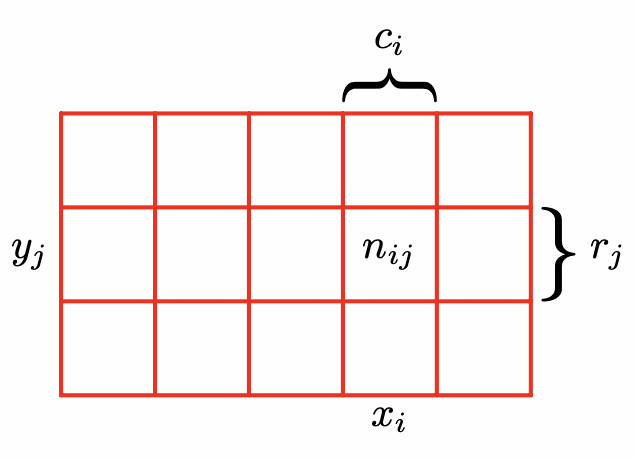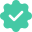# Rules of Probability Theory Explained

posted in | Feb 02, 2023 | 698 views

A key concept in the field of machine learning is that of uncertainty. It arises both through noise on measurements, as well as through the finite size of data sets. Probability theory provides a consistent framework for the quantification and manipulation of uncertainty and forms one of the central foundations for pattern recognition. When combined with decision theory, it allows us to make optimal predictions given all the information available to us, even though that information may be incomplete or ambiguous.I will introduce the basic concepts of probability theory by considering a simple example. Imagine we have two boxes, one red and one blue, and in the red box we have 2 apples and 6 oranges, and in the blue box we have 3 apples and 1 orange as illustrated in the figure beside. Now suppose we randomly pick one of the boxes and from that box we randomly select an item of fruit, and having observed which sort of fruit it is we replace it in the box from which it came. We could imagine repeating this process many times. Let us suppose that in so doing we pick the red box 40% of the time and we pick the blue box 60% of the time, and that when we remove an item of fruit from a box we are equally likely to select any of the pieces of fruit in the box.In this example, the identity of the box that will be chosen is a random variable, which we shall denote by $B$. This random variable can take one of two possible values, namely $r$ (corresponding to the red box) or $b$ (corresponding to the blue box). Similarly, the identity of the fruit is also a random variable and will be denoted by $F$ . It can take either of the values $a$ (for apple) or $o$ (for orange).

To begin with, we shall define the probability of an event to be the fraction of times that event occurs out of the total number of trials, in the limit that the total number of trials goes to infinity. Thus the probability of selecting the red box is $4/10$ and the probability of selecting the blue box is $6/10$. We write these probabilities as $p(B = r) = 4/10$ and $p(B = b) = 6/10$. Note that, by definition, probabilities must lie in the interval $\left[0, 1\right]$. Also, if the events are mutually exclusive and if they include all possible outcomes (for instance, in this example the box must be either red or blue), then we see that the probabilities for those events must sum to one.

We can now ask questions such as: “what is the overall probability that the selection procedure will pick an apple?”, or “given that we have chosen an orange, what is the probability that the box we chose was the blue one?”. We can answer questions such as these, and indeed much more complex questions associated with problems in pattern recognition, once we have equipped ourselves with the two elementary rules of probability, known as the sum rule and the product rule. Having obtained these rules, we shall then return to our boxes of fruit example.In order to derive the rules of probability, consider the slightly more general example shown in figure beside involving two random variables $X$ and $Y$ (which could for instance be the Box and Fruit variables considered above). We shall suppose that $X$ can take any of the values $x_i$ where $i = 1,\dots,M$, and $Y$ can take the values $y_j$ where $j = 1,\dots,L$. Consider a total of $N$ trials in which we sample both of the variables $X$ and $Y$,and let the number of such trials in which $X=x_i$ and $Y =y_j$ be $n_{ij}$. Also, let the number of trials in which $X$ takes the value $x_i$ (irrespective of the value that $Y$ takes) be denoted by $c_i$, and similarly let the number of trials in which $Y$ takes the value $y_j$ be denoted by $r_j$.

The probability that X will take the value $x_i$ and $Y$ will take the value $y_j$ is written $p(X = x_i, Y = y_j)$ and is called the joint probability of $X = x_i$ and $Y = y_j$. It is given by the number of points falling in the cell $i$, $j$ as a fraction of the total number of points, and hence

$$p(X = x_i, Y = y_j) = \frac{n_{ij}}{N}$$

Here we are implicitly considering the limit $N → \infty$. Similarly, the probability that $X$ takes the value $x_i$ irrespective of the value of $Y$ is written as $p(X = x_i)$ and is given by the fraction of the total number of points that fall in column $i$, so that

$$p(X = x_i) = \frac{c_i}{N}$$

Because the number of instances in column $i$ in our figure is just the sum of the number of instances in each cell of that column, we have $c_i = \sum_{j} n_{ij}$ and therefore, from both the above equations, we have

$$p(X = x_i) = \sum_{j=1}^{L} p(X = x_i, Y = y_j)$$

which is the sum rule of probability. Note that $p(X = x_i)$ is sometimes called the marginal probability, because it is obtained by marginalizing, or summing out, the other variables (in this case $Y$).

If we consider only those instances for which $X = x_i$, then the fraction of such instances for which $Y = y_j$ is written $p(Y = y_j | X = x_i)$ and is called the conditional probability of $Y = y_j$ given $X = x_i$. It is obtained by finding the fraction of those points in column i that fall in cell $i$ ,$j$ and hence is given by

$$p(Y = y_j | X = x_i) = \frac{n_{ij}}{c_i}$$

and finally from the first, second, and the fourth equations, we can then derive the following relationship

\begin{aligned} p(X = x_i | Y = y_j ) &= \frac{n_{ij}}{N} = \frac{n_{ij}}{c_i} \cdot \frac{c_i}{N} \\ &= p(Y = y_j | X = x_i)p(X=x_i) \end{aligned}

which is the product rule of probability.

We can derive the sum and product rules of probability by considering two random variables, $X$, which takes the values $\{ x_i \}$ where $i = 1,...,M$, and $Y$, which takes the values $\{y_j\}$ where $j = 1,...,L$. In the second illustration provided, we have $M = 5$ and $L = 3$. If we consider a total number $N$ of instances of these variables, then we denote the number of instances where $X = x_i$ and $Y = y_j$ by $n_{ij}$, which is the number of $y_j$ points in the corresponding cell of the array. The number of points in column $i$, corresponding to $X = x_i$, is denoted by $c_i$, and the number of points in row $j$, corresponding to $Y = y_j$, is denoted by $r_j$.

## The rules of probability

So far we have been quite careful to make a distinction between a random variable, such as the box $B$ in the fruit example, and the values that the random variable can take, for example $r$ if the box were the red one. Thus the probability that $B$ takes the value $r$ is denoted $p(B = r)$. Although this helps to avoid ambiguity, it leads to a rather cumbersome notation, and in many cases there will be no need for such pedantry. Instead, we may simply write $p(B)$ to denote a distribution over the random variable $B$, or $p(r)$ to denote the distribution evaluated for the particular value $r$, provided that the interpretation is clear from the context.

With this more compact notation, we can write the two fundamental rules of probability theory in the following form.

\begin{aligned} \textbf{Sum rule} \;\;\;\;\;\;\;\; & p(X) = \sum_{Y} p(X, Y) \\ \textbf{Product rule} \;\;\;\;\;\;\;\; & p(X, Y ) = p(Y |X )p(X )\end{aligned}

Here $p(X, Y )$ is a joint probability and is verbalized as “the probability of $X$ and $Y$”. Similarly, the quantity $p(Y |X)$ is a conditional probability and is verbalized as “the probability of $Y$ given $X$”, whereas the quantity $p(X)$ is a marginal probability and is simply “the probability of $X$”. These two simple rules form the basis for all of the probabilistic machinery.

## bonus: Bayes' theorem

From the product rule, together with the symmetry property $p(X, Y ) = p(Y, X)$, we immediately obtain the following relationship between conditional probabilities

$$p(Y |X) = \frac{p(X|Y )p(Y )}{p(X )}$$

which is called Bayes’ theorem and which plays a central role in pattern recognition and machine learning. Using the sum rule, the denominator in Bayes’ theorem can be expressed in terms of the quantities appearing in the numerator

$$p(X) =  p(X|Y )p(Y )$$

We can view the denominator in Bayes’ theorem as being the normalization constant required to ensure that the sum of the conditional probability on the left-hand side of equation over all values of $Y$ equals one.

That's it! You now know the sum and the product rules of probability as well as the Bayes' theorem. Don't stop here. Go explore more. Here are some related topics you might wanna take a look at:

• Joint Distributions
• Probability Densities
• Expectations and Covariances

Until next time!

https://shi.foo/rss/priyansh on Feb 04, 2023 Example comment on the first article. This comment also demonstrates the ability to format text and insert code with auto-highlight like: class ProbabilityRules: @staticmethod def sum_rule(p_XY): p_X = {} for X, Y in p_XY: p_X[X] = p_X.get(X, 0) + p_XY[(X, Y)] return p_X @staticmethod def product_rule(p_Y_given_X, p_X): p_XY = {} for Y, X in p_Y_given_X: p_XY[(X, Y)] = p_Y_given_X[(Y, X)] * p_X[X] return p_XY @staticmethod def bayes_theorem(p_X_given_Y, p_Y, p_X): p_Y_given_X = {} for X, Y in p_X_given_Y: p_Y_given_X[(Y, X)] = (p_X_given_Y[(X, Y)] * p_Y[Y]) / p_X[X] return p_Y_given_XAlso, I can add formulae by wrapping them in a single or double '\$' symbols: $$p(X = x_i) = \sum_{j=1}^{L} p(X = x_i, Y = y_j)$$